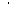# MINLPLib

### A Library of Mixed-Integer and Continuous Nonlinear Programming Instances

#### Instance st_e33

 Formatsⓘ ams gms lp mod nl osil pip py Primal Bounds (infeas ≤ 1e-08)ⓘ -400.00000000 p1 ( gdx sol ) (infeas: 3e-14) Other points (infeas > 1e-08)ⓘ Dual Boundsⓘ -400.00000040 (ANTIGONE)-400.00000040 (BARON)-400.00000000 (COUENNE)-400.00000000 (GUROBI)-400.00000000 (LINDO)-400.00000000 (SCIP) Referencesⓘ Tawarmalani, M and Sahinidis, N V, Convexification and Global Optimization in Continuous and Mixed-Integer Nonlinear Programming: Theory, Algorithms, Software, and Applications, Kluwer, 2002.Xia, Q, A constrained problem difficult for genetic algorithms, 1996. Sourceⓘ BARON book instance misc/e33 Added to libraryⓘ 03 Sep 2002 Problem typeⓘ QCP #Variablesⓘ 9 #Binary Variablesⓘ 0 #Integer Variablesⓘ 0 #Nonlinear Variablesⓘ 3 #Nonlinear Binary Variablesⓘ 0 #Nonlinear Integer Variablesⓘ 0 Objective Senseⓘ min Objective typeⓘ linear Objective curvatureⓘ linear #Nonzeros in Objectiveⓘ 6 #Nonlinear Nonzeros in Objectiveⓘ 0 #Constraintsⓘ 6 #Linear Constraintsⓘ 3 #Quadratic Constraintsⓘ 3 #Polynomial Constraintsⓘ 0 #Signomial Constraintsⓘ 0 #General Nonlinear Constraintsⓘ 0 Operands in Gen. Nonlin. Functionsⓘ Constraints curvatureⓘ indefinite #Nonzeros in Jacobianⓘ 23 #Nonlinear Nonzeros in Jacobianⓘ 7 #Nonzeros in (Upper-Left) Hessian of Lagrangianⓘ 4 #Nonzeros in Diagonal of Hessian of Lagrangianⓘ 0 #Blocks in Hessian of Lagrangianⓘ 1 Minimal blocksize in Hessian of Lagrangianⓘ 3 Maximal blocksize in Hessian of Lagrangianⓘ 3 Average blocksize in Hessian of Lagrangianⓘ 3.0 #Semicontinuitiesⓘ 0 #Nonlinear Semicontinuitiesⓘ 0 #SOS type 1ⓘ 0 #SOS type 2ⓘ 0 Minimal coefficientⓘ 1.0000e-02 Maximal coefficientⓘ 1.6000e+01 Infeasibility of initial pointⓘ 0 Sparsity JacobianⓘSparsity Hessian of Lagrangianⓘ```\$offlisting
*
*  Equation counts
*      Total        E        G        L        N        X        C        B
*          7        5        0        2        0        0        0        0
*
*  Variable counts
*                   x        b        i      s1s      s2s       sc       si
*      Total     cont   binary  integer     sos1     sos2    scont     sint
*         10       10        0        0        0        0        0        0
*  FX      0
*
*  Nonzero counts
*      Total    const       NL      DLL
*         30       23        7        0
*
*  Solve m using NLP minimizing objvar;

Variables  x1,x2,x3,x4,x5,x6,x7,x8,x9,objvar;

Positive Variables  x1,x2,x3,x4,x5,x6,x7,x8;

Equations  e1,e2,e3,e4,e5,e6,e7;

e1..    x1 + x2 - x3 - x4 =E= 0;

e2.. -x9*(x3 + x4) + 0.03*x1 + 0.01*x2 =E= 0;

e3..    x3 - x5 + x6 =E= 0;

e4..    x4 + x7 - x8 =E= 0;

e5.. x9*x3 - 0.025*x5 + 0.02*x6 =L= 0;

e6.. x9*x4 + 0.02*x7 - 0.015*x8 =L= 0;

e7..  - 6*x1 - 16*x2 + 9*x5 - 10*x6 - 10*x7 + 15*x8 + objvar =E= 0;

* set non-default bounds
x1.up = 300;
x2.up = 300;
x3.up = 100;
x4.up = 200;
x5.up = 100;
x6.up = 300;
x7.up = 100;
x8.up = 200;
x9.lo = 0.01; x9.up = 0.03;

Model m / all /;

m.limrow=0; m.limcol=0;
m.tolproj=0.0;

\$if NOT '%gams.u1%' == '' \$include '%gams.u1%'

\$if not set NLP \$set NLP NLP
Solve m using %NLP% minimizing objvar;

```

Last updated: 2023-08-16 Git hash: 2519540e